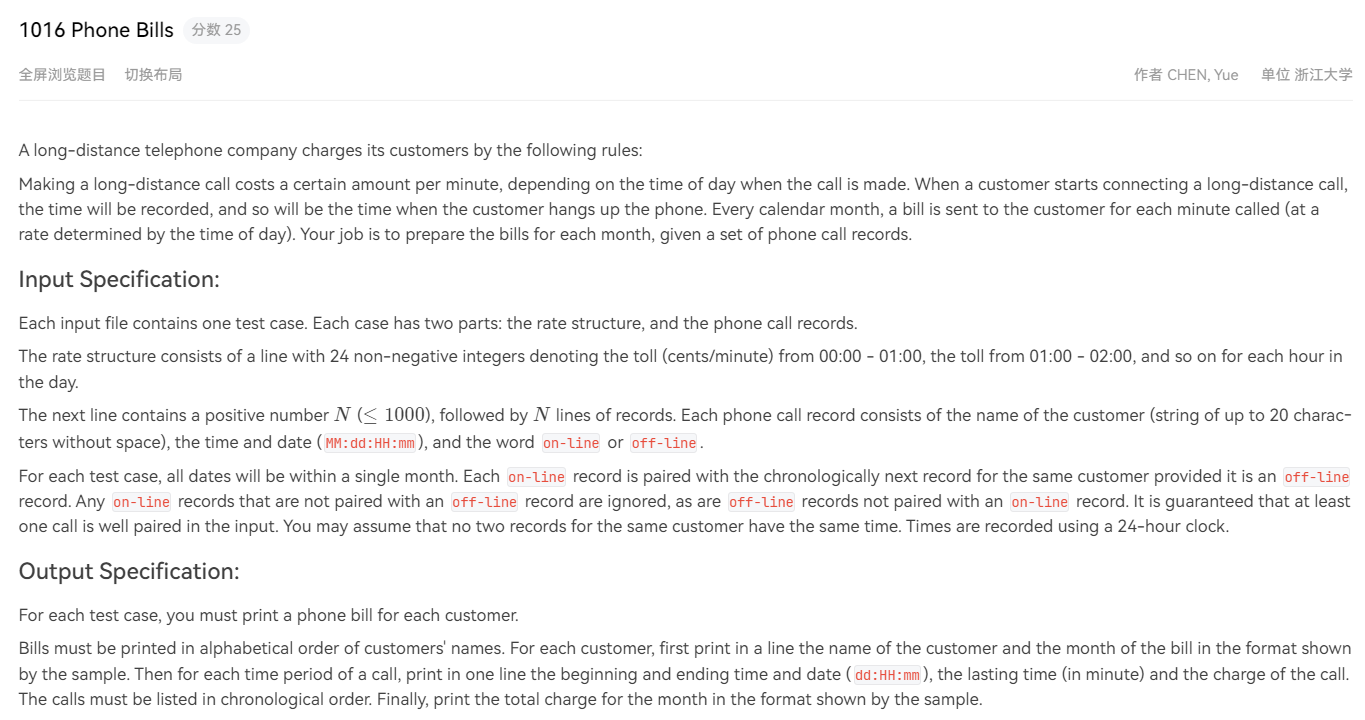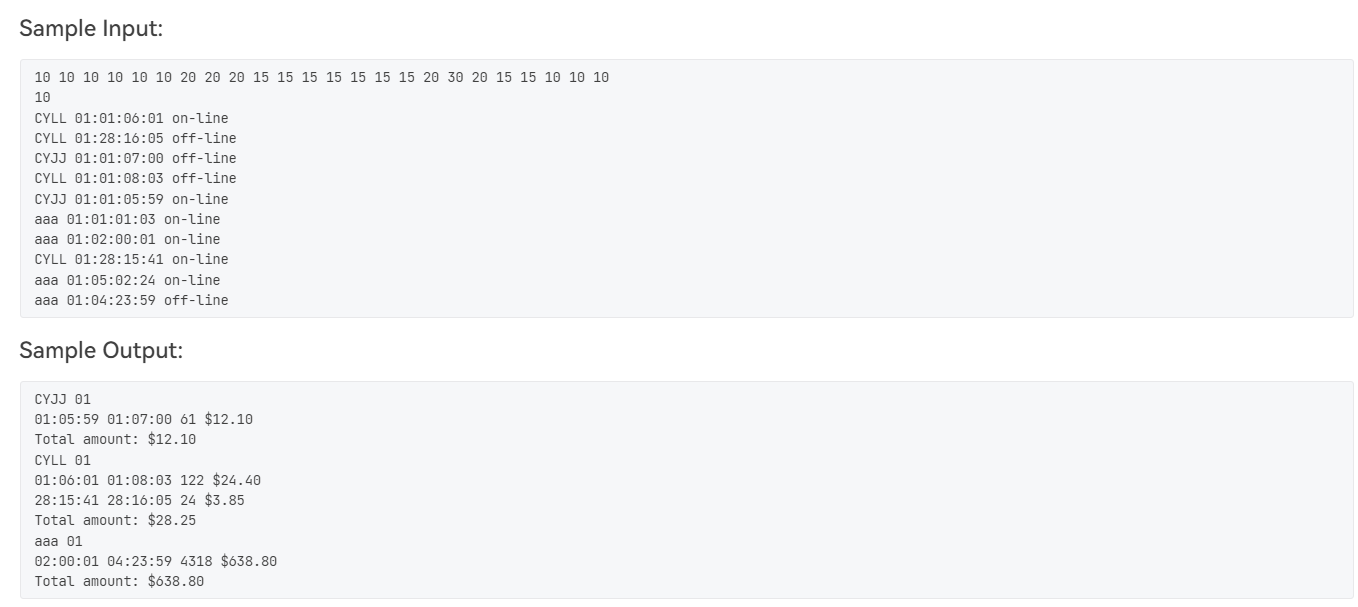# 题目# 解题思路

• 本题对时间的处理，都是以分钟为单位（老套路了，对时间的处理，要么换成秒，要么换成分钟，），比如01：01：22，表示当月1号1点22分，那就换成（1 24 60+1 * 60 + 22），是以每个月1号0点0分为基准，这样处理很方便。我们用sum_time来存这个数值，也方便后面对记录排序，同时也处理了通话记录跨天的问题。比如当月1号23点55分——当月2号0点3分，我们的处理方式可以很好的解决这一问题。
• 电话费的问题我们依旧是相同的思路，即对每一条通话记录的时间计算其费用，例如 `CYLL 01:01:06:01 on-line `这条记录，我们依然以当月0点0分为基准，对其进行费率计算。先算分钟的费率+天数的费率，再算小时的费率，本例就是6点到7点的费率 分钟 + 一天费率的总和 60 * 几号 +每个小时对应的费率的和。然后讲两个匹配的记录的费用和做差即可算出当前通话的费用。最后单位问题，题目给的是美分，要求输出美元，所以最后需要转换。

# 实现代码

``````#include<iostream>
#include<cstdio>
#include<vector>
#include<cstring>
#include<map>
#include<sstream>
#include<algorithm>
using namespace std;

typedef struct node
{
string str;
//status存储通话记录状态，0表示off-line，1表示on-line
int month,day,hour,minute,sum_time,status;
}NODE;

bool cmp(NODE a,NODE b)
{
//升序排列
return a.str != b.str ? a.str < b.str : a.sum_time < b.sum_time;
}

//计算每个通话记录的费率
double bill(NODE a, int *price)
{
double total = price[a.hour] * a.minute + price * 60 * a.day;

for(int i=0;i<a.hour;i++)
{
total+=price[i]*60;
}

}

int price;

int main()
{
#ifdef ONLINE_JUDGE
#else
freopen("1.txt", "r", stdin);
#endif

int temp=0;
for(int i=0;i<24;i++)
{
cin>>price[i];
temp+=price[i];
}
price=temp;

int num;
cin>>num;

vector<NODE> v(num);

for(int i=0;i<num;i++)
{
string temp;
cin>>v[i].str;
scanf("%d:%d:%d:%d",&v[i].month,&v[i].day,&v[i].hour,&v[i].minute);

v[i].sum_time = v[i].day*24*60 + v[i].hour*60 + v[i].minute;

cin>>temp;
temp == "on-line" ? v[i].status=1 : v[i].status=0;
}

sort(v.begin(),v.end(),cmp);

map<string,vector<NODE>> mmap;

for(int i=1;i<num;i++)
{
if(v[i].str == v[i-1].str && v[i].status==0 && v[i-1].status==1)
{
mmap[v[i-1].str].push_back(v[i-1]);
mmap[v[i].str].push_back(v[i]);
}
}

map<string,vector<NODE>>::iterator iter;
for( iter = mmap.begin() ; iter != mmap.end() ; iter++ )
{
cout<<iter->first;
printf(" %02d\n",iter->second.month);

double temp=0.0;
for(int i=1;i<iter->second.size();i++,i++)
{
double total_price=bill(iter->second[i],price)-bill(iter->second[i-1],price);

printf("%02d:%02d:%02d %02d:%02d:%02d %d \$%.2lf\n",iter->second[i-1].day,iter->second[i-1].hour,iter->second[i-1].minute,iter->second[i].day,iter->second[i].hour,iter->second[i].minute,iter->second[i].sum_time-iter->second[i-1].sum_time,total_price);

temp+=total_price;
}
printf("Total amount: \$%.2lf\n",temp);
}

return 0;
}``````

lionの金库